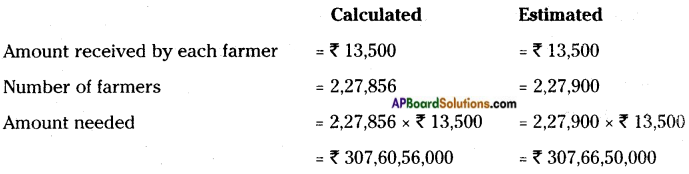# AP Board 6th Class Maths Solutions Chapter 1 Numbers All Around us Unit Exercise

AP State Syllabus AP Board 6th Class Maths Solutions Chapter 1 Numbers All Around us Unit Exercise Textbook Questions and Answers.

## AP State Syllabus 6th Class Maths Solutions 1st Lesson Numbers All Around us Unit Exercise

Question 1.
Write each of the following in numeral form.
i) Hundred crores hundred thousands and hundred.
Indian system: 100,01,00,100.

ii) Twenty billion four hundred ninety seven million pinety six thousands four hundred seventy two.
20,497,096,472Question 2.
Write each of the following in words in both Hindu-Arabic and International system.
i) 8275678960
ii) 5724500327
iii) 1234567890
i) 8275678960
Hindu – Arabic system: 827,56,78,960
Eight hundred twenty seven crores fifty six lakhs seventy eight thousand nine hundred and sixty.
International system: 8,275,678,960
Eight billion two hundred seventy five million six hundred seventy eight thousand nine hundred and sixty.

ii) Hindu-Arabic system: 572,45,00,327
Five hundred seventy two crores forty five lakhs three hundred and twenty seven. International system: 5,724,500,327
Five billion seven hundred twenty four million five hundred thousand three hundred and twenty seven,

iii) 1234567890
Hindu-Arabic system: 123,45,67,890
One hundred twenty three crores forty five lakhs sixty seven thousand eight hundred and ninety.
International system: 1,234,567,890
One billion two hundred thirty four million five hundred sixty seven thousand eight hundred and ninety.

Question 3.
Find the difference between the place values of the two eight’s in 98978056.
Place values of 8 in Hindu-Arabic system of the given number are 8,000 and 80,00,000
Difference = 80,00,000 – 8,000 = 79,92,000
Place values of 8 in International system of the given number are 8000 and 8,000,000
Difference = 8,000,000 – 8,000 = 79,92,000Question 4.
How many 6 digit numbers are there in all?
Number of 6 digit numbers = greatest 6 digit number – greatest 5 digit number
= 9,99,999 – 99,999 = 9,00,000.

Question 5.
How many thousands make one million?
1000 thousands can make one million.
1 Million = 1000 Thousands.

Question 6.
Collect ‘5’ mobile numbers and arrange them in ascending and descending order.
Let the 5 mobile numbers are: 9247568320, 9849197602, 8125646682, 6305481954, 7702177046
Ascending order: 6305481954, 7702177046, 8125646682, 9247568320, 9849197602
Descending older: 9849197602, 9247568320, 8125646682, 7702177046, 6305481954

Question 7.
Pravali has one sister and one brother. Pravali’s father earned one million rupees and wanted to distribute the amount equally. Estimate approximate amount each will get in lakhs and verify with actual division.
One million = 10,00,000 = 10 lakhs
Pravali’s father distributed 10 lakhs amount to his 3 children equally.
So, the share of each children = 10 lakhs ÷ 3 = Rs. 3,33,333
= Rs. 3,00,000 (approximately)

Question 8.
Government wants to distribute Rs. 13,500 to each farmer. There are 2,27,856 farmers in a district. Calculate the total amount needed for that district. (First estimate, then calculate)Question 9.
Explain terms Cusec, T.M.C, Metric tonne, Kilometer.
a) Cusec: A unit of flow equal to 1 cubic foot per second.
1 Cusec = 0.028316 cubic feet per second = 28.316 litre per second.
Cusec is the unit to measure the liquids in large numbers quantity.

b) TMC: TMC is the unit to measure the water in large quantity.
TMC means Thousand Million Cubic feet.
1 TMC = 0.28316000000 litre
= 28.316 billion litre
= 2831.6 crores litre

c) Metric tonne: Metric tonne is the unit of weight.
Metric tonne = 1000 kg = 10 quintals
We should use this unit in measuring crops, paddy, dall, etc.

d) Kilometer: Kilometre is the unit of length.
1 kilometer = 1000 meters.
We should use this unit is measuring distance between villages, towns, cities,…. etc.